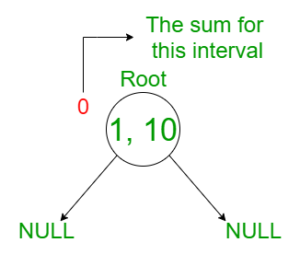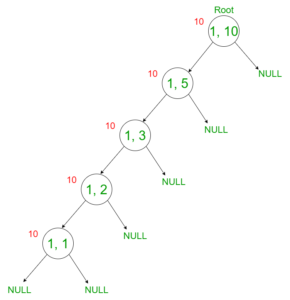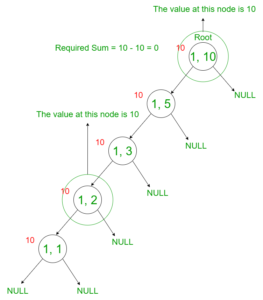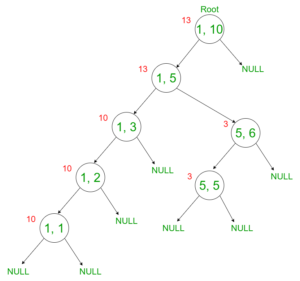Related Articles

# Dynamic Segment Trees : Online Queries for Range Sum with Point Updates

• Difficulty Level : Expert
• Last Updated : 13 Aug, 2021

Prerequisites: Segment Tree
Given a number N which represents the size of the array initialized to 0 and Q queries to process where there are two types of queries:

1. 1 P V: Put the value V at position P.
2. 2 L R: Output the sum of values from L to R.

Constraints:

• 1 ≤ N ≤ 1018
• Q ≤ 105
• 1 ≤ L ≤ R≤ N

Note: Queries are online. Therefore:

• L = (previousAnswer + L) % N + 1
• R = (previousAnswer + R) % N + 1

Examples:

Input: N = 5, Q = 5, arr[][] = {{1, 2, 3}, {1, 1, 4}, {1, 3, 5}, {1, 4, 7}, {2, 3, 4}}
Output: 12
Explanation:
There are five queries. Since N = 5, therefore, initially, the array is {0, 0, 0, 0, 0}
For query 1: 1 2 3 array = {0, 3, 0, 0, 0}
For query 2: 1 1 4 array = {4, 3, 0, 0, 0}
For query 3: 1 3 5 array = {4, 3, 5, 0, 0}
For query 4: 1 4 7 array = {4, 3, 5, 7, 0}
For query 5: 2 3 4 Sum from [3, 4] = 7 + 5 = 12.

Input: N = 3, Q = 2, arr[][] = {{1, 1, 1}, {1, 2, 2}, {1, 3, 3}}
Output: 0

Approach: Here, since the updates are high, Kadane’s algorithm doesn’t work quite well. Moreover, since it is given that the queries are online, a simple segment tree would not be able to solve this problem because the constraints for the number of elements is very high. Therefore, a new type of data structure, a dynamic segment tree is used in this problem.
Dynamic Segment Tree: Dynamic segment tree is not a new data structure. It is very similar to the segment tree. The following are the properties of the dynamic segment tree:

• Instead of using an array to represent the intervals, a node is created whenever a new interval is to be updated.
• The following is the structure of the node of the dynamic segment tree:

## C++

 `// Every node contains the value and``// the left subtree and right subtree``struct` `Node {``    ``long` `long` `value;``    ``struct` `Node *L, *R;``};``  ` `struct` `Node* getnode()``{``    ``struct` `Node* temp = ``new` `struct` `Node;``    ``temp->value = 0;``    ``temp->L = NULL;``    ``temp->R = NULL;``    ``return` `temp;``}`
• Clearly, the above structure is the same as a Binary Search Tree. In every node, we are storing the node’s value and two pointers pointing to the left and right subtree.
• The Interval of the root is from [1, N], the interval of the left subtree will be [1, N/2] and the interval for the right subtree will be [N/2 + 1, N].
• Similarly, for every node, we can calculate the interval it is representing. Let’s say the interval of the current node is [L, R]. Then, the Interval of its left and right subtree are [L, (L + R)/2] and [(L + R)/2+1, R] respectively.
• Since we are creating a new node only when required, the build() function from the segment tree is completely removed.

Before getting into the algorithm for the operations, let’s define the terms used in this article:

• Node’s interval: It is the interval the node is representing.
• Required interval: Interval for which the sum is to calculate.
• Required index: Index at which Update is required.

The following is the algorithm used for the operations on the tree with the above-mentioned properties:

1. Point Update: The following algorithm is used for the point update:
2. If the interval at the node doesn’t overlap with the required index then return.
3. If the node is a NULL entry then create a new node with the appropriate intervals and descend into that node by going back to step 2 for every new child created.
4. If both, intervals and the index at which the value is to be stored are equal, then store the value into at that node.
5. If the interval at the node overlaps partially with the required index then descend into its children and continue the execution from step 2.
2. Finding the sum for every query: The following algorithm is used to find the sum for every query:
2. If the node is a NULL or the interval at that node doesn’t overlap with the required interval, then return 0.
3. If the interval at the node completely overlaps with the required interval then return the value stored at the node.
4. If the interval at the node overlaps partially with the required interval then descend into its children and continue the execution from step 2 for both of its children.

Example: Lets visualize the update and sum with an example. Let N = 10 and the operations needed to perform on the tree are as follows:

1. Insert 10 at position 1.
2. Find the sum of value of indices from 2 to 8.
3. Insert 3 at position 5.
4. Find the sum of value of indices from 3 to 6.
• Initially, for the value N = 10, the tree is empty. Therefore:• Insert 10 at position 1. In order to do this, create a new node until we get the required interval. Therefore:• Find the sum of value of indices from 2 to 8. In order to do this, the sum from [1, 8] is found and the value [1, 2] is subtracted from it. Since the node [1, 8] is not yet created, the value of [1, 8] is the value of the root [1, 10]. Therefore:• Insert 3 at position 5. In order to do this, create a new node until we get the required interval. Therefore:Below is the implementation of the above approach:

## C++

 `// C++ program for the implementation``// of the Dynamic segment tree and``// perform the range updates on the``// given queries` `#include ` `using` `namespace` `std;``typedef` `long` `long` `ll;` `// Structure of the node``struct` `Node {` `    ``ll value;``    ``struct` `Node *L, *R;``};` `// Structure to get the newly formed``// node``struct` `Node* getnode()``{``    ``struct` `Node* temp = ``new` `struct` `Node;``    ``temp->value = 0;``    ``temp->L = NULL;``    ``temp->R = NULL;``    ``return` `temp;``}` `// Creating the Root node``struct` `Node* root;` `// Function to perform the point update``// on the dynamic segment tree``void` `UpdateHelper(``struct` `Node* curr, ll index,``                  ``ll L, ll R, ll val)``{` `    ``// If the index is not overlapping``    ``// with the index``    ``if` `(L > index || R < index)``        ``return``;` `    ``// If the index is completely overlapping``    ``// with the index``    ``if` `(L == R && L == index) {` `        ``// Update the value of the node``        ``// to the given value``        ``curr->value = val;``        ``return``;``    ``}` `    ``// Computing the middle index if none``    ``// of the above base cases are satisfied``    ``ll mid = L - (L - R) / 2;``    ``ll sum1 = 0, sum2 = 0;` `    ``// If the index is in the left subtree``    ``if` `(index <= mid) {` `        ``// Create a new node if the left``        ``// subtree is is null``        ``if` `(curr->L == NULL)``            ``curr->L = getnode();` `        ``// Recursively call the function``        ``// for the left subtree``        ``UpdateHelper(curr->L, index, L, mid, val);``    ``}` `    ``// If the index is in the right subtree``    ``else` `{` `        ``// Create a new node if the right``        ``// subtree is is null``        ``if` `(curr->R == NULL)``            ``curr->R = getnode();` `        ``// Recursively call the function``        ``// for the right subtree``        ``UpdateHelper(curr->R, index, mid + 1, R, val);``    ``}` `    ``// Storing the sum of the left subtree``    ``if` `(curr->L)``        ``sum1 = curr->L->value;` `    ``// Storing the sum of the right subtree``    ``if` `(curr->R)``        ``sum2 = curr->R->value;` `    ``// Storing the sum of the children into``    ``// the node's value``    ``curr->value = sum1 + sum2;``    ``return``;``}` `// Function to find the sum of the``// values given by the range``ll queryHelper(``struct` `Node* curr, ll a,``               ``ll b, ll L, ll R)``{` `    ``// Return 0 if the root is null``    ``if` `(curr == NULL)``        ``return` `0;` `    ``// If the index is not overlapping``    ``// with the index, then the node``    ``// is not created. So sum is 0``    ``if` `(L > b || R < a)``        ``return` `0;` `    ``// If the index is completely overlapping``    ``// with the index, return the node's value``    ``if` `(L >= a && R <= b)``        ``return` `curr->value;` `    ``ll mid = L - (L - R) / 2;` `    ``// Return the sum of values stored``    ``// at the node's children``    ``return` `queryHelper(curr->L, a, b, L, mid)``           ``+ queryHelper(curr->R, a, b, mid + 1, R);``}` `// Function to call the queryHelper``// function to find the sum for``// the query``ll query(``int` `L, ``int` `R)``{``    ``return` `queryHelper(root, L, R, 1, 10);``}` `// Function to call the UpdateHelper``// function for the point update``void` `update(``int` `index, ``int` `value)``{``    ``UpdateHelper(root, index, 1, 10, value);``}` `// Function to perform the operations``// on the tree``void` `operations()``{``    ``// Creating an empty tree``    ``root = getnode();` `    ``// Update the value at position 1 to 10``    ``update(1, 10);` `    ``// Update the value at position 3 to 5``    ``update(3, 5);` `    ``// Finding sum for the range [2, 8]``    ``cout << query(2, 8) << endl;` `    ``// Finding sum for the range [1, 10]``    ``cout << query(1, 10) << endl;` `}` `// Driver code``int` `main()``{``    ``operations();` `    ``return` `0;``}`

## Java

 `// Java program for the implementation``// of the Dynamic segment tree and``// perform the range updates on the``// given queries` `class` `GFG {` `    ``// Structure of the node``    ``static` `class` `Node {``        ``int` `value;``        ``Node L, R;` `    ``}` `    ``// Structure to get the newly formed``    ``// node``    ``static` `Node getnode() {``        ``Node temp = ``new` `Node();``        ``temp.value = ``0``;``        ``temp.L = ``null``;``        ``temp.R = ``null``;``        ``return` `temp;``    ``}` `    ``// Creating the Root node``    ``static` `Node root = ``new` `Node();` `    ``// Function to perform the point update``    ``// on the dynamic segment tree``    ``static` `void` `UpdateHelper(Node curr, ``int` `index, ``int` `L, ``int` `R, ``int` `val) {` `        ``// If the index is not overlapping``        ``// with the index``        ``if` `(L > index || R < index)``            ``return``;` `        ``// If the index is completely overlapping``        ``// with the index``        ``if` `(L == R && L == index) {` `            ``// Update the value of the node``            ``// to the given value``            ``curr.value = val;``            ``return``;``        ``}` `        ``// Computing the middle index if none``        ``// of the above base cases are satisfied``        ``int` `mid = L - (L - R) / ``2``;``        ``int` `sum1 = ``0``, sum2 = ``0``;` `        ``// If the index is in the left subtree``        ``if` `(index <= mid) {` `            ``// Create a new node if the left``            ``// subtree is is null``            ``if` `(curr.L == ``null``)``                ``curr.L = getnode();` `            ``// Recursively call the function``            ``// for the left subtree``            ``UpdateHelper(curr.L, index, L, mid, val);``        ``}` `        ``// If the index is in the right subtree``        ``else` `{` `            ``// Create a new node if the right``            ``// subtree is is null``            ``if` `(curr.R == ``null``)``                ``curr.R = getnode();` `            ``// Recursively call the function``            ``// for the right subtree``            ``UpdateHelper(curr.R, index, mid + ``1``, R, val);``        ``}` `        ``// Storing the sum of the left subtree``        ``if` `(curr.L != ``null``)``            ``sum1 = curr.L.value;` `        ``// Storing the sum of the right subtree``        ``if` `(curr.R != ``null``)``            ``sum2 = curr.R.value;` `        ``// Storing the sum of the children into``        ``// the node's value``        ``curr.value = sum1 + sum2;``        ``return``;``    ``}` `    ``// Function to find the sum of the``    ``// values given by the range``    ``static` `int` `queryHelper(Node curr, ``int` `a, ``int` `b, ``int` `L, ``int` `R) {` `        ``// Return 0 if the root is null``        ``if` `(curr == ``null``)``            ``return` `0``;` `        ``// If the index is not overlapping``        ``// with the index, then the node``        ``// is not created. So sum is 0``        ``if` `(L > b || R < a)``            ``return` `0``;` `        ``// If the index is completely overlapping``        ``// with the index, return the node's value``        ``if` `(L >= a && R <= b)``            ``return` `curr.value;` `        ``int` `mid = L - (L - R) / ``2``;` `        ``// Return the sum of values stored``        ``// at the node's children``        ``return` `queryHelper(curr.L, a, b, L, mid) + queryHelper(curr.R, a, b, mid + ``1``, R);``    ``}` `    ``// Function to call the queryHelper``    ``// function to find the sum for``    ``// the query``    ``static` `int` `query(``int` `L, ``int` `R) {``        ``return` `queryHelper(root, L, R, ``1``, ``10``);``    ``}` `    ``// Function to call the UpdateHelper``    ``// function for the point update``    ``static` `void` `update(``int` `index, ``int` `value) {``        ``UpdateHelper(root, index, ``1``, ``10``, value);``    ``}` `    ``// Function to perform the operations``    ``// on the tree``    ``static` `void` `operations() {``        ``// Creating an empty tree``        ``root = getnode();` `        ``// Update the value at position 1 to 10``        ``update(``1``, ``10``);` `        ``// Update the value at position 3 to 5``        ``update(``3``, ``5``);` `        ``// Finding sum for the range [2, 8]``        ``System.out.println(query(``2``, ``8``));` `        ``// Finding sum for the range [1, 10]``        ``System.out.println(query(``1``, ``10``));` `    ``}` `    ``// Driver code``    ``public` `static` `void` `main(String[] args) {``        ``operations();``    ``}``}` `// This code is contributed by sanjeev2552`

## Javascript

 ``
Output:
```5
15```

Time Complexity: O(Q * logN)
Auxiliary Space: O(N)

Attention reader! Don’t stop learning now. Get hold of all the important DSA concepts with the DSA Self Paced Course at a student-friendly price and become industry ready.  To complete your preparation from learning a language to DS Algo and many more,  please refer Complete Interview Preparation Course.

In case you wish to attend live classes with experts, please refer DSA Live Classes for Working Professionals and Competitive Programming Live for Students.

My Personal Notes arrow_drop_up